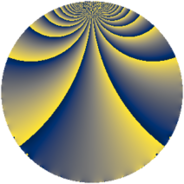Properties

 Label 656.1.hLevel $656$ Weight $1$ Character orbit 656.h Rep. character $\chi_{656}(655,\cdot)$ Character field $\Q$ Dimension $4$ Newform subspaces $1$ Sturm bound $84$ Trace bound $0$

Related objects

Defining parameters

 Level: $$N$$ $$=$$ $$656 = 2^{4} \cdot 41$$ Weight: $$k$$ $$=$$ $$1$$ Character orbit: $$[\chi]$$ $$=$$ 656.h (of order $$2$$ and degree $$1$$) Character conductor: $$\operatorname{cond}(\chi)$$ $$=$$ $$164$$ Character field: $$\Q$$ Newform subspaces: $$1$$ Sturm bound: $$84$$ Trace bound: $$0$$

Dimensions

The following table gives the dimensions of various subspaces of $$M_{1}(656, [\chi])$$.

Total New Old
Modular forms 19 4 15
Cusp forms 13 4 9
Eisenstein series 6 0 6

The following table gives the dimensions of subspaces with specified projective image type.

$$D_n$$ $$A_4$$ $$S_4$$ $$A_5$$
Dimension 4 0 0 0

Trace form

 $$4 q + 4 q^{9} + O(q^{10})$$ $$4 q + 4 q^{9} + 4 q^{25} - 4 q^{41} - 8 q^{45} + 4 q^{49} - 8 q^{57} - 8 q^{77} + 4 q^{81} + O(q^{100})$$

Decomposition of $$S_{1}^{\mathrm{new}}(656, [\chi])$$ into newform subspaces

Label Dim. $$A$$ Field Image CM RM Traces $q$-expansion
$a_{2}$ $a_{3}$ $a_{5}$ $a_{7}$
656.1.h.a $4$ $0.327$ $$\Q(\zeta_{16})^+$$ $D_{8}$ $$\Q(\sqrt{-41})$$ None $$0$$ $$0$$ $$0$$ $$0$$ $$q-\beta _{1}q^{3}-\beta _{2}q^{5}-\beta _{3}q^{7}+(1+\beta _{2}+\cdots)q^{9}+\cdots$$

Decomposition of $$S_{1}^{\mathrm{old}}(656, [\chi])$$ into lower level spaces

$$S_{1}^{\mathrm{old}}(656, [\chi]) \cong$$ $$S_{1}^{\mathrm{new}}(164, [\chi])$$$$^{\oplus 3}$$